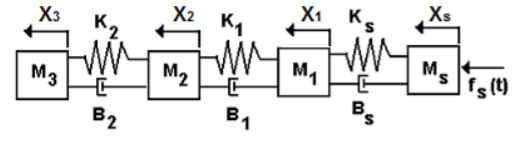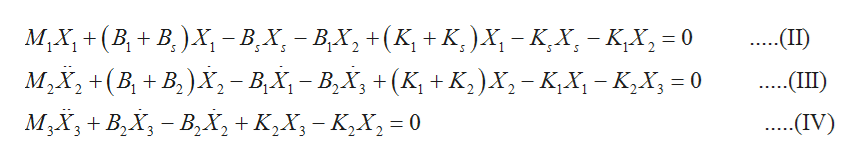Question
76 views

Four masses are connected with spring mass dampers. x1, x2, x3 and xs are the horizontal displacements
of the masses. Derive the equation of motion of the systemhelp_outlineImage TranscriptioncloseXs Х1 к. X2 Хз WИ МИ м. fs (t) M1 M2 Мз В. ВА B2 fullscreen
check_circle

Step 1

For the system to be in equilibrium, the sum of the horizontal forces on each mass is 0.

Step 2

By balancing the horizontal forces of mass Ms we get,

Step 3

Similarly by balancing the horizontal forces of masses M1...help_outlineImage TranscriptioncloseМ,х, +(В, + В.)х, -В. х, — В.Х, + (к, +к)х, - кх, - кх,3D0 M2X2+(BB)X- B,X- BX (K K,)X,-K,X- K2X 0 MXBBX, + K2X-K,X,=0 .(II) (KK2) X2-KX- K2X = 0 ..(Ш) (IV) fullscreen

### Want to see the full answer?

See Solution

#### Want to see this answer and more?

Solutions are written by subject experts who are available 24/7. Questions are typically answered within 1 hour.*

See Solution
*Response times may vary by subject and question.
Tagged in